# 实验2

x1,y1=1.2,3.57
x2,y2=2.26,8.7

print('{:-^40}'.format('输出1'))
print('x1={},y1={}'.format(x1,y1))
print('x2={},y2={}'.format(x2,y2))

print('{:-^40}'.format('输出2'))
print('x1 = {:.1f}, y1 = {:.1f}'.format(x1, y1))
print('x2 = {:.1f}, y2 = {:.1f}'.format(x2, y2))

print('{:-^40}'.format('输出3'))
print('x1 = {:<15}, y1 = {:<15}'.format(x1, y1))
print('x2 = {:<15}, y2 = {:<15}'.format(x2, y2))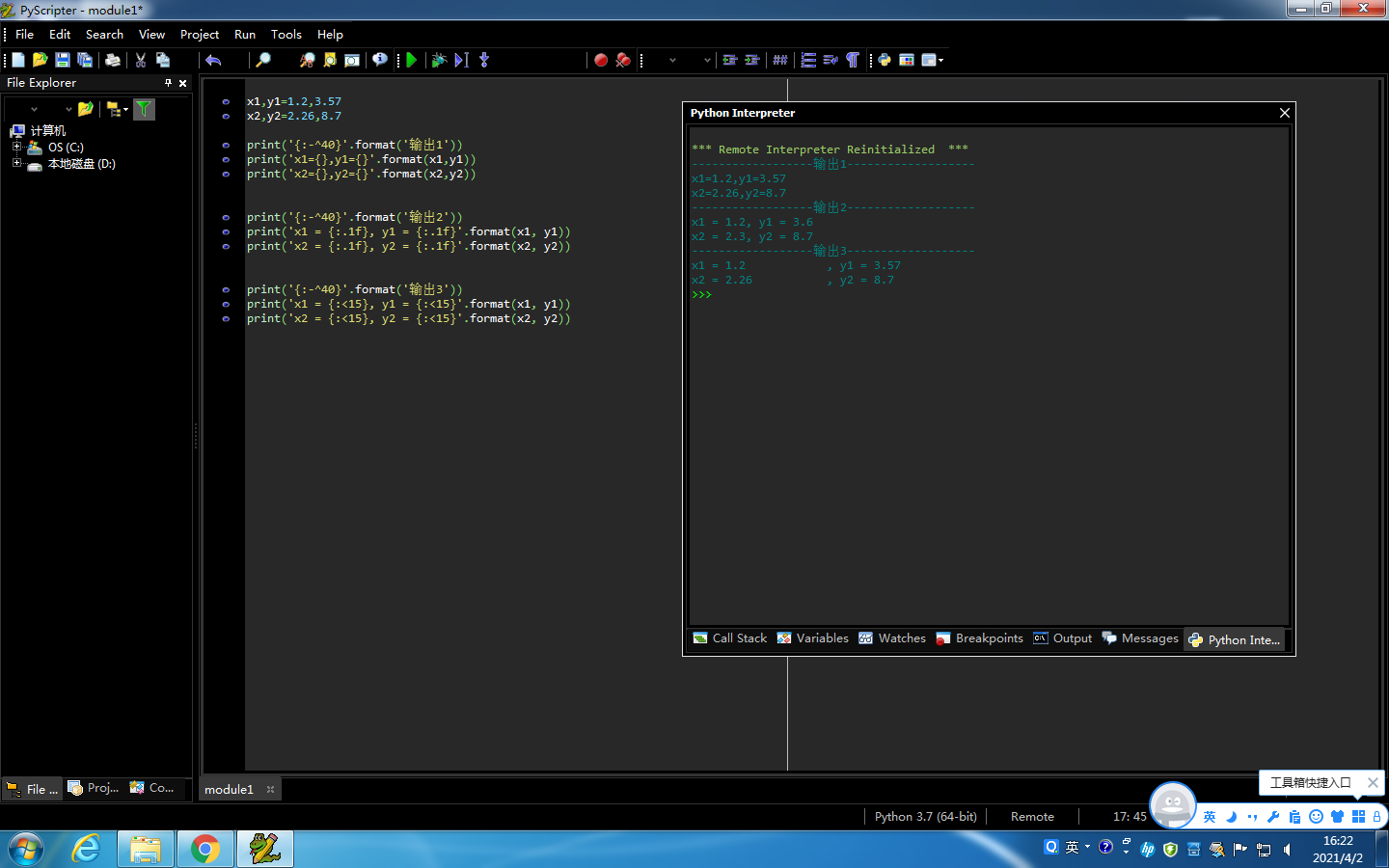s=(input('输入一个字符串：'))
s=s.replace('a','')
print(s.upper())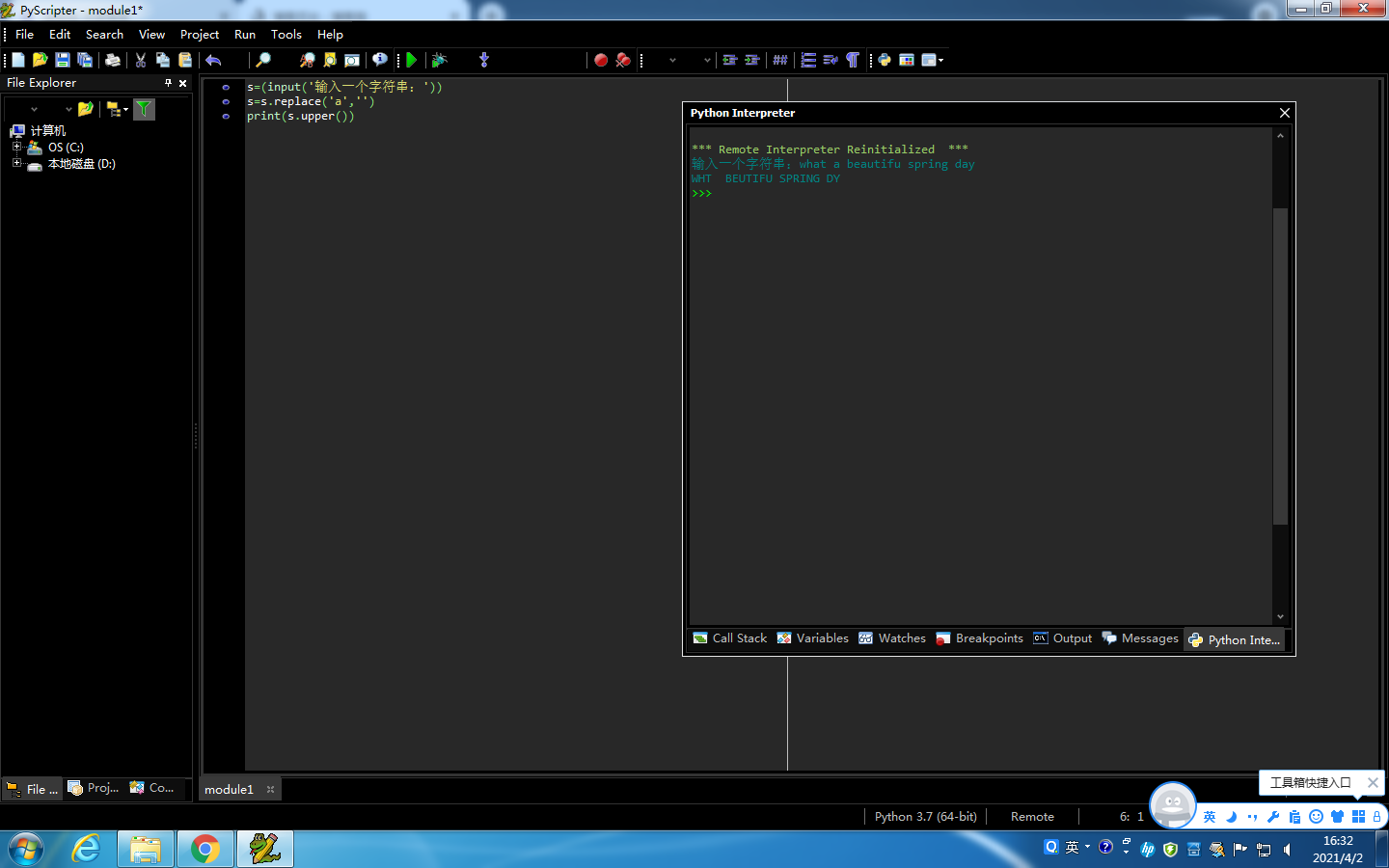name_list=['david bowie','louis armstrong','leonard cohen','bob dylan','cocteau twins']

n=1
for name in name_list:
print(f'{n}:{name.title()}')
n+=1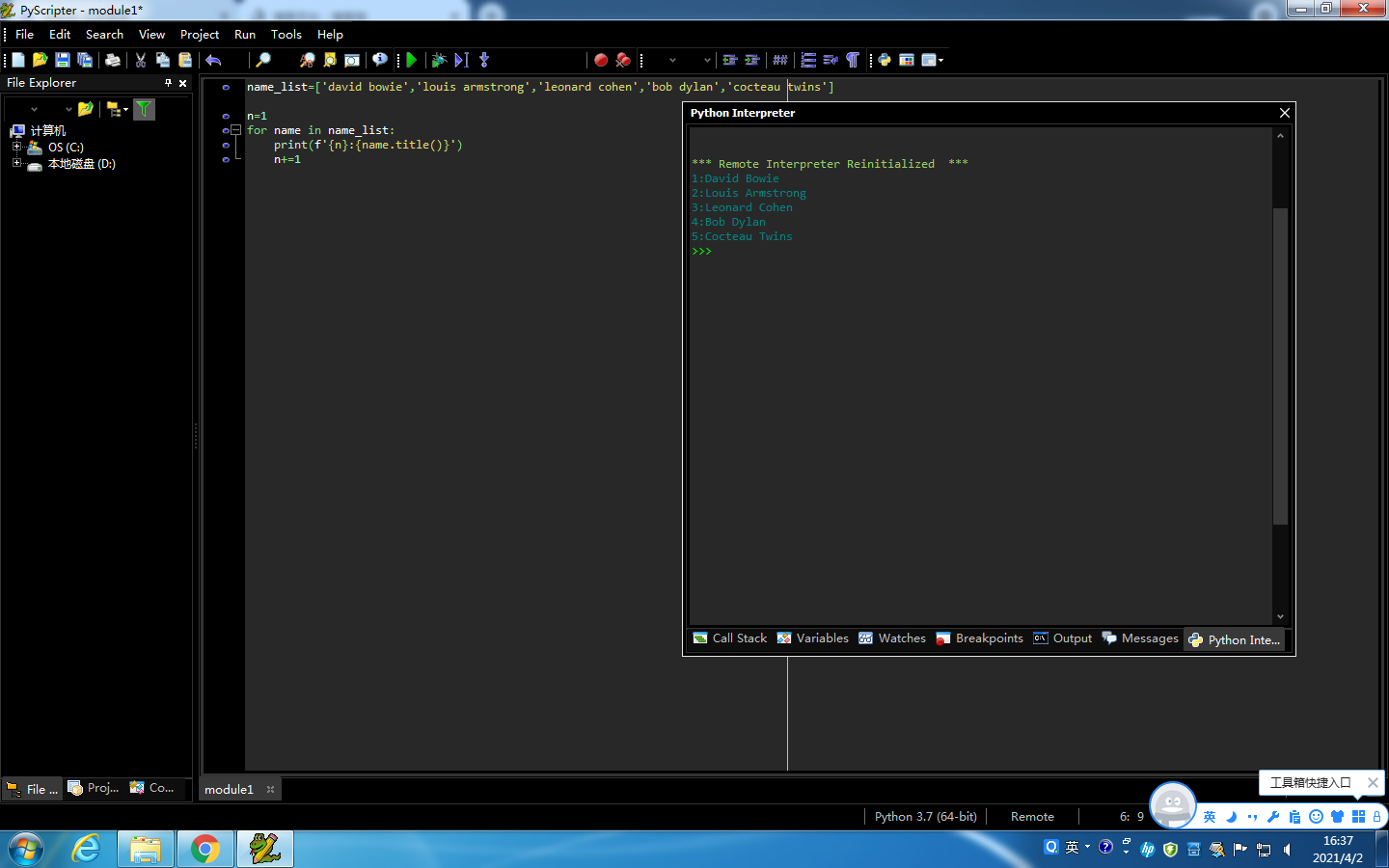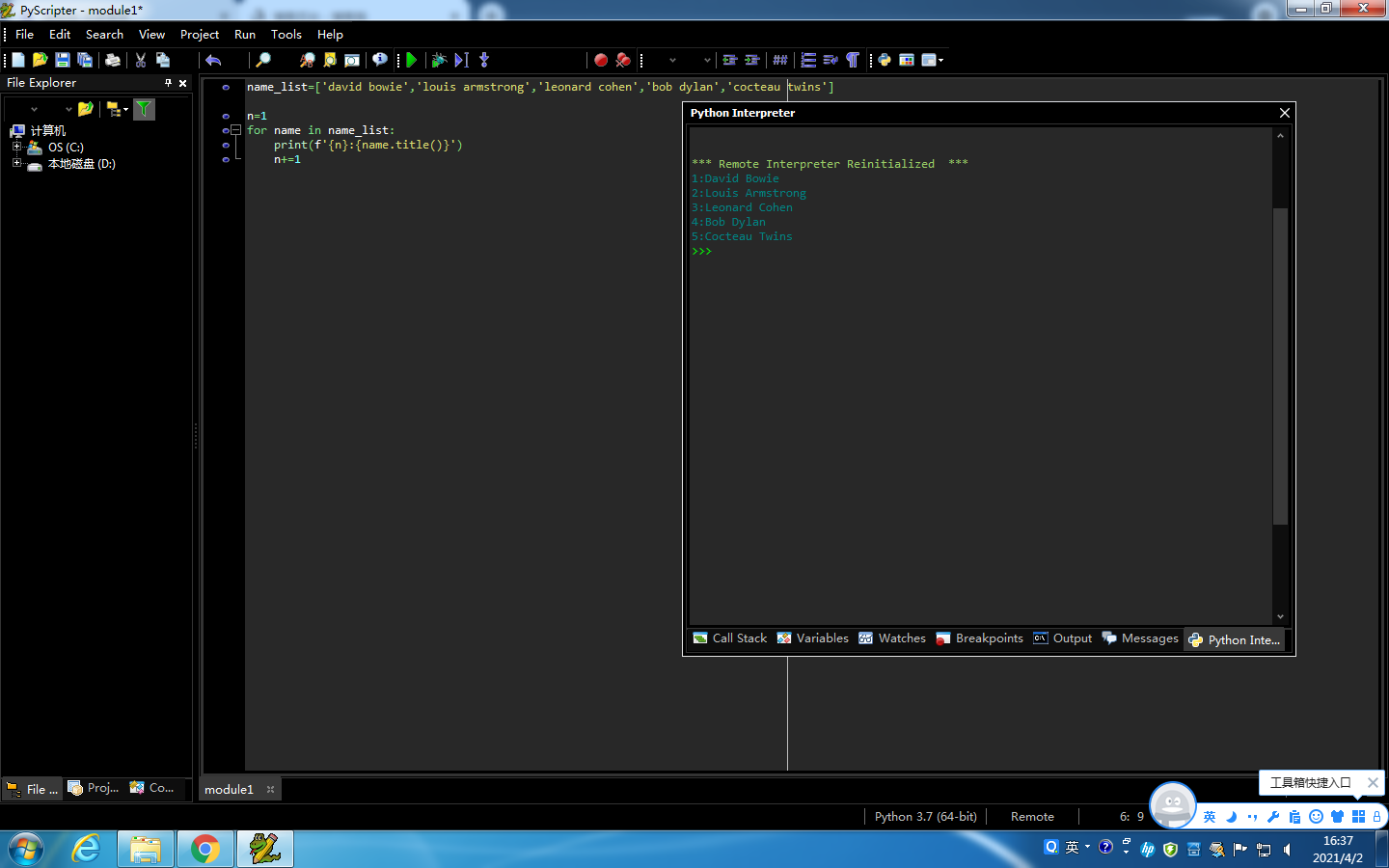name_list=['david bowie','louis armstrong','leonard cohen','bob dylan','cocteau twins']
name_list.sort()
n=1
for name in name_list:
print(f'{n}.{name.title()}')
n+=1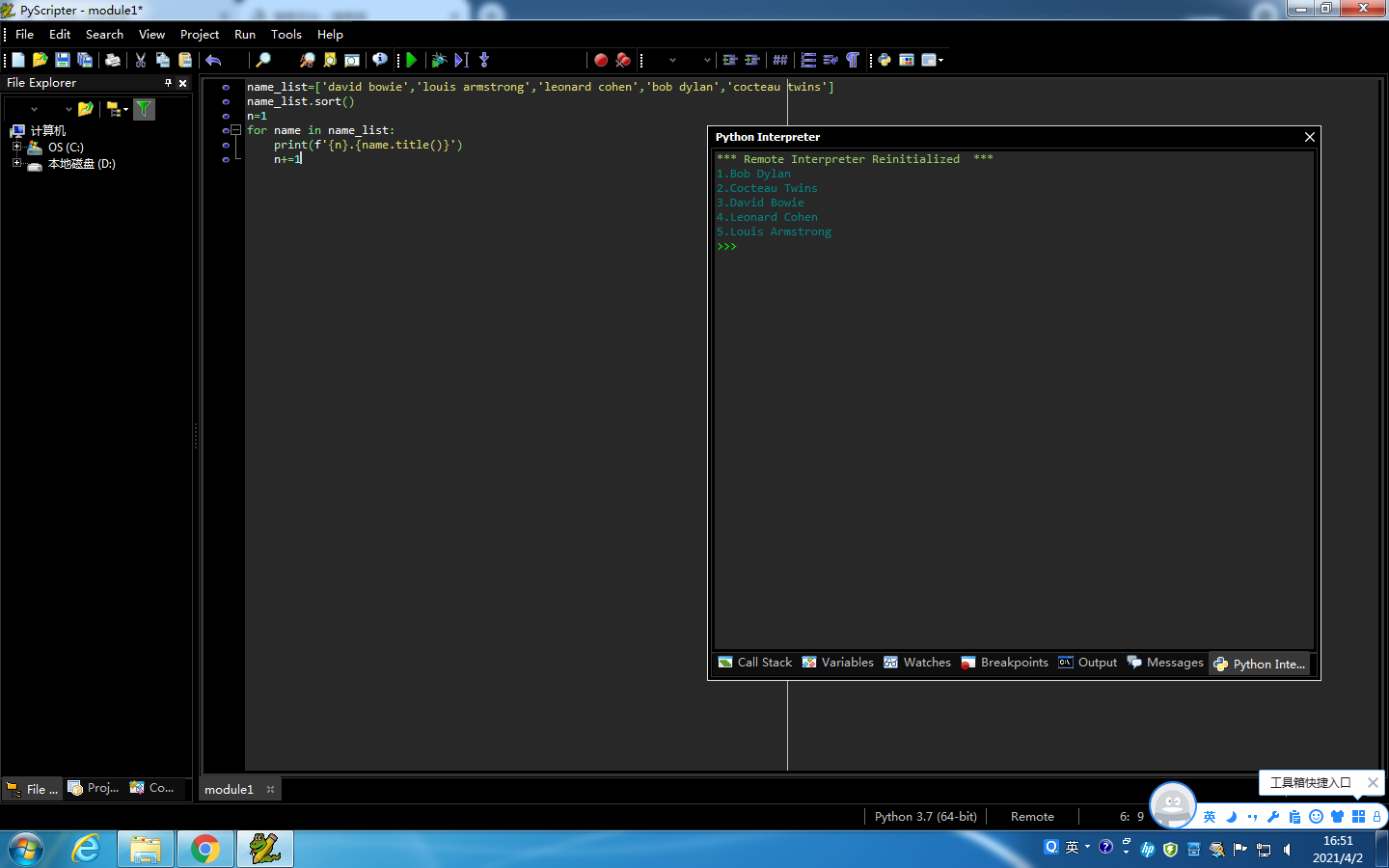text='''
The Zen of Python, by Tim Peters

Beautiful is better than ugly.
Explicit is better than implicit.
Simple is better than complex.
Complex is better than complicated.
Flat is better than nested.
Sparse is better than dense.
Readability counts.
Special cases aren't special enough to break the rules.
Although practicality beats purity.
Errors should never pass silently.
Unless explicitly silenced.
In the face of ambiguity, refuse the temptation to guess.
There should be one-- and preferably only one --obvious way to do it.
Although that way may not be obvious at first unless you're Dutch.
Now is better than never.
Although never is often better than *right* now.
If the implementation is hard to explain, it's a bad idea.
If the implementation is easy to explain, it may be a good idea.
Namespaces are one honking great idea -- let's do more of those!
'''

print(len(text.splitlines()))
print(len(text.split()))
print(len(text))
print(text.count(""))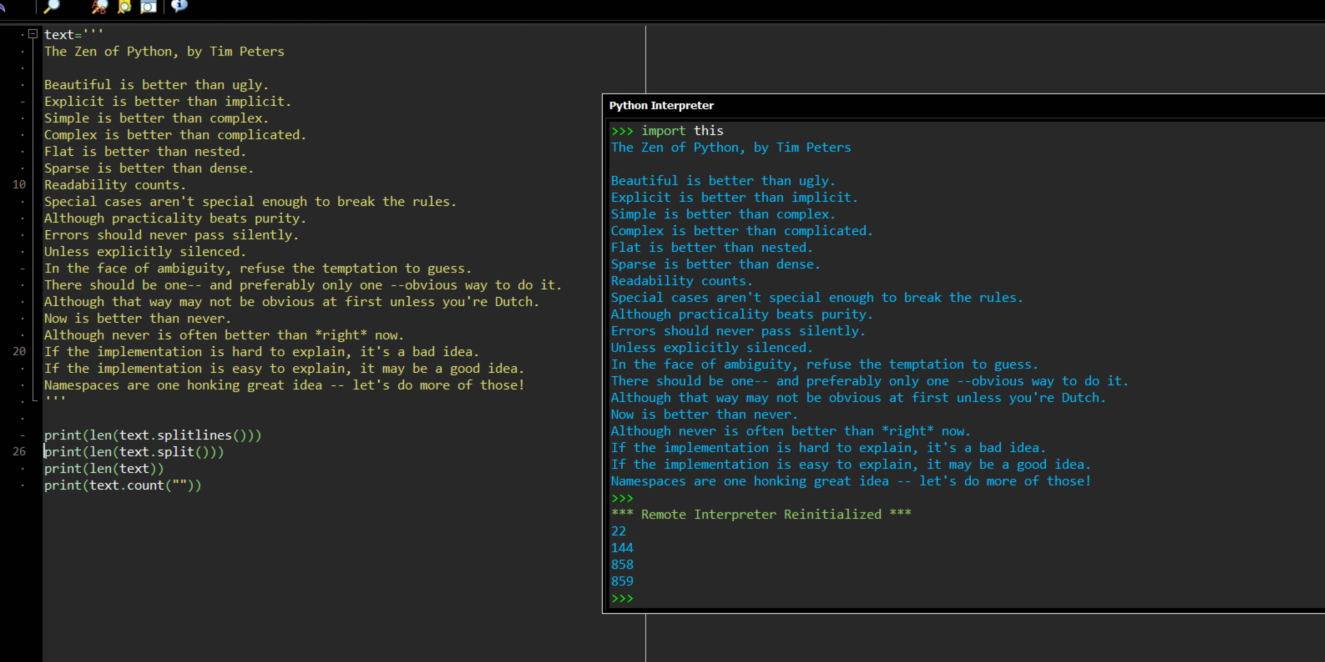x = list({})
for n in range(3):
a = input("输入想要加入愿望清单的事：")
x.append(a)
print("{:-^50}".format("我的愿望清单"))

for n in range(1,4):
print(n,end="")
print("."+x[n-1])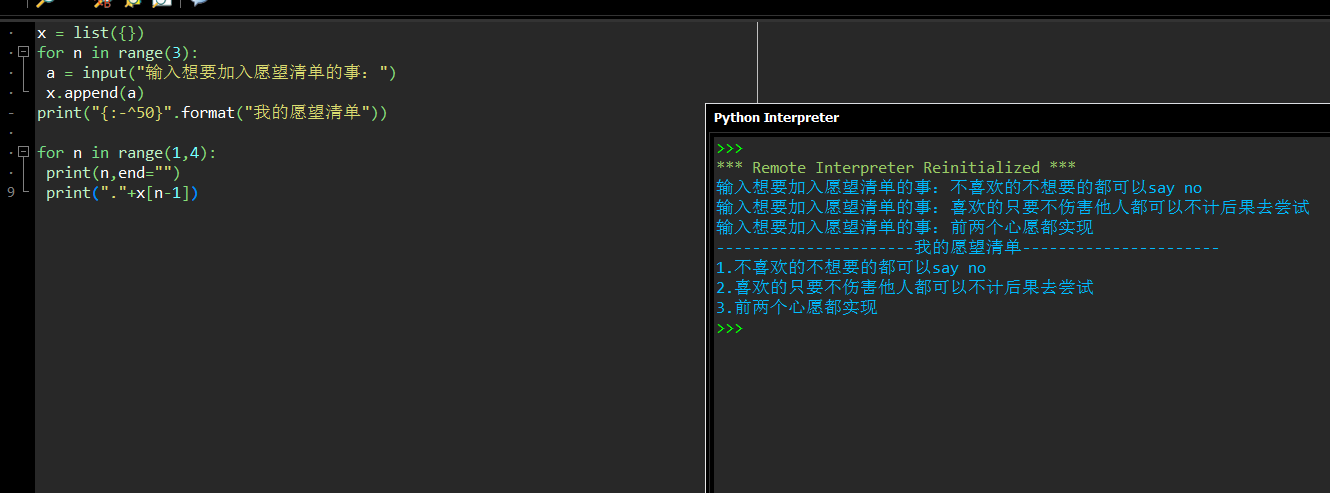posted @ 2021-04-08 14:41  是花花吖  阅读(11)  评论(0编辑  收藏